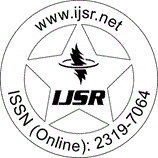International Journal of Science and Research (IJSR)
Call for Papers | Fully Refereed | Open Access | Double Blind Peer Reviewed

Research Paper | Aerospace Engineering | Iraq | Volume 7 Issue 12, December 2018

# Partial Integral Equations

Alaa Hussein Khaleel

Abstract: In this paper we give a study of the partial integral equations and discuss the eigenvalue problems related to the linear partial integral equations. Also we use the collection and Galerkin's methods to find an approximated solution for the eigenvalue problems related to the linear partial integral equations.

Keywords: partial integral equations, integral equations, collection methods, Galerkin's methods.

Edition: Volume 7 Issue 12, December 2018,

Pages: 1245 - 1248